## 5.10 Memory update

26.1903GPPAdaptive Multi-Rate - Wideband (AMR-WB) speech codecSpeech codec speech processing functionsTranscoding functionsTS

An update of the states of the synthesis and weighting filters is needed in order to compute the target signal in the next subframe.

After the two gains have been quantized, the excitation signal, u(n), in the present subframe is found by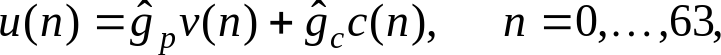( 56)

where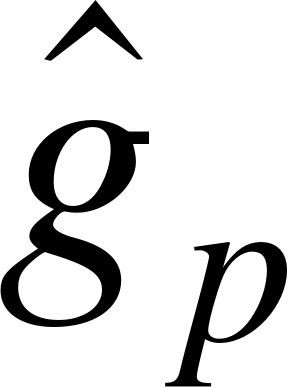and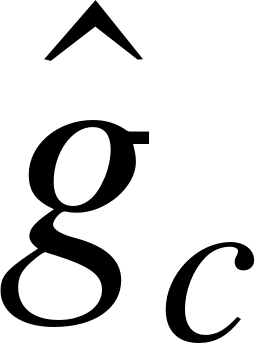are the quantized adaptive and fixed codebook gains, respectively, vi(n) the adaptive codebook vector (interpolated past excitation), and c(n) is the fixed codebook vector (algebraic code including pitch sharpening). The states of the filters can be updated by filtering the signal r(n)u(n) (difference between residual and excitation) through the filters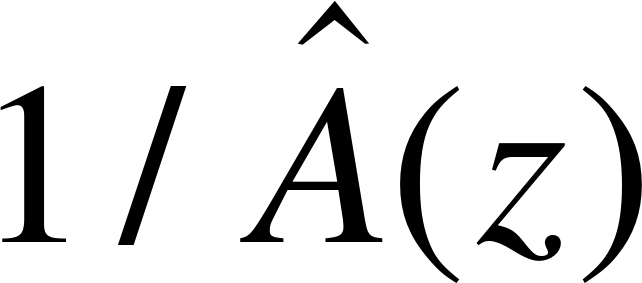andfor the 64 sample subframe and saving the states of the filters. This would require 3 filterings. A simpler approach which requires only one filtering is as follows. The local synthesis speech,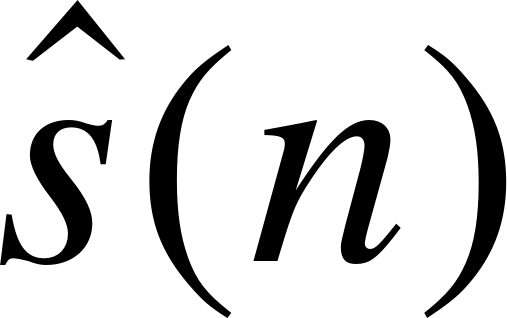, is computed by filtering the excitation signal through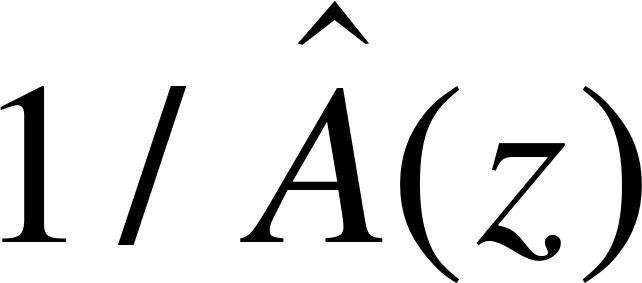. The output of the filter due to the input r(n)u(n) is equivalent to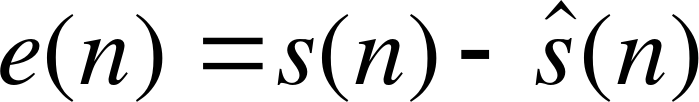. So the states of the synthesis filter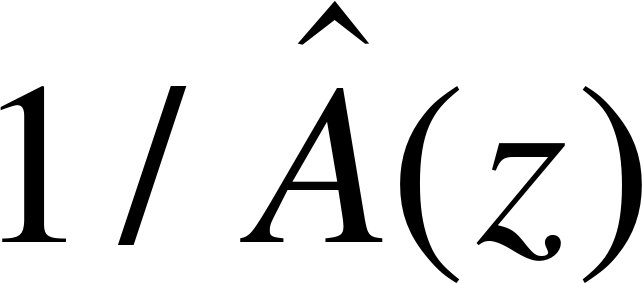are given by e(n),n=48,…,63. Updating the states of the filtercan be done by filtering the error signal e(n) through this filter to find the perceptually weighted error ew(n). However, the signal ew(n) can be equivalently found by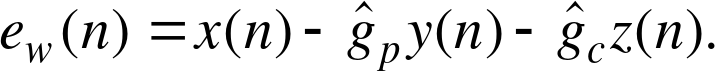( 57)

Since the signals x(n), y(n), and z(n) are available, the states of the weighting filter are updated by computing ew(n) as in Equation (54) for. This saves two filterings.